Most Affordable JEE | NEET | 8,9,10 Preparation by Kota's Top IITian Doctor Faculties

# Combine Translational and Rotational Motion | Physics 11, JEE & NEET`
Here you will study about rolling without slipping Motion and Combine Translational and Rotational Motion.
Combine Translational and Rotational Motion If in case of rotational motion of a body about a fixed axis (called spining) the axis of rotation also moves, the motion is called combined translatory and rotatory motion. Motion of rolling wheel and flying saucer can be examples of this type of motion. Total K.E. $\quad \mathrm{E}_{\mathrm{T}}=\mathrm{E}_{\mathrm{t}}+\mathrm{E}_{\mathrm{r}}$ $=\frac{1}{2} \mathrm{mv}_{\mathrm{cm}}^{2}+\frac{1}{2} \mathrm{I} \omega^{2} \quad \mathrm{v}_{\mathrm{cm}}=$ velocity of $\mathrm{c} . \mathrm{m} .$ of the body In this case translational kinetic energy $\left(E_{t}\right)$ and rotational kinetic energy $\left(E_{r}\right)$ does not dependent on each other. Rolling without slipping: If the object rolls across a surface in such a manner that there is no relative motion of object and surface at the point of contact, this type of motion is known as Rolling without slipping As rotation produces translation, so $\quad v=r \omega, \quad a=r \alpha$ Total kinetic energy $\mathrm{E}_{\mathrm{T}}=\mathrm{E}_{\mathrm{t}}+\mathrm{E}_{\mathrm{t}}$ So $\quad \mathrm{E}_{\mathrm{T}}=\frac{1}{2} \mathrm{Mv}^{2}+\frac{1}{2} \mathrm{I} \omega^{2}=\frac{1}{2} \mathrm{M} \omega^{2} \mathrm{r}^{2}+\frac{1}{2} \mathrm{I} \omega^{2}=\frac{1}{2} \omega^{2}\left(\mathrm{I}+\mathrm{Mr}^{2}\right)$ $\left.=\frac{1}{2} \frac{v^{2}}{r^{2}} \cdot\left(M K^{2}+M r^{2}\right) \quad \text { (since } I=M K^{2}\right)$ $\mathrm{E}_{\mathrm{T}}=\frac{1}{2} \mathrm{M}\left(1+\frac{\mathrm{K}^{2}}{\mathrm{r}^{2}}\right) \mathrm{v}^{2}$ $\mathrm{E}_{\mathrm{t}}=\frac{1}{2} \mathrm{Mv}^{2} \quad$ and $\quad \mathrm{E}_{\mathrm{r}}=\frac{1}{2} \mathrm{M} \frac{\mathrm{K}^{2}}{\mathrm{r}^{2}} \mathrm{v}^{2}$ $\mathbf{E}_{\mathrm{t}}: \mathbf{E}_{\mathrm{r}}: \mathbf{E}_{\mathrm{T}}=1: \frac{\mathrm{K}^{2}}{\mathrm{R}^{2}}: 1+\frac{\mathrm{K}^{2}}{\mathrm{R}^{2}}$ Friction is responsible for the rolling motion but there is no loss of energy against friction since there is no relative motion between body and surface at point of contact. Ex. A body of mass $M$ and radius $r$, rolling on a smooth horizontal floor with velocity $v$, rolls up an irregular inclined plane up to a vertical height $\left(3 v^{2} / 4 g\right)$. Compute the moment of inertia of the body and comment on its shape? The total kinetic energy of the body- \begin{array}{l}{\mathrm{E}=\mathrm{E}_{\mathrm{t}}+\mathrm{E}_{\mathrm{r}}=\frac{1}{2} \mathrm{Mv}^{2}+\frac{1}{2} \mathrm{I} \omega^{2}} \\ {\text { or } \quad \mathrm{E}=\frac{1}{2} \mathrm{Mv}^{2}\left[1+\left(\mathrm{I} / \mathrm{Mr}^{2}\right)\right]} & { \text { [as }\mathrm{v}=\mathrm{r} \omega]}\end{array}   When it rolls up a irregular inclined plane of height $h\left(3 v^{2} / 4 g\right),$ its $K E$ is converted into $P E,$ so by conservation of mechanical energy $\frac{1}{2} \mathrm{Mv}^{2}\left[1+\frac{\mathrm{I}}{\mathrm{Mr}^{2}}\right]=\mathrm{Mg}\left[\frac{3 \mathrm{v}^{2}}{4 \mathrm{g}}\right] \quad$ which on simplification gives $\mathrm{I}=(1 / 2) \mathrm{Mr}^{2} .$ This result clearly indicates that the body is either a disc or cylinder.     ROLLING ON A PLANE SURFACE: $\# \quad v_{A}=2 V_{\mathrm{cm}}, \quad V_{\mathrm{B}}=0, V_{\mathrm{C}}=V_{\mathrm{cm}}$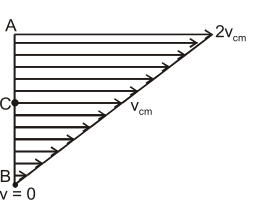Upper point has twice the velocity of CM. Rolling motion for which $\mathrm{V}_{\mathrm{c}}=0$ is called pure rotating motion Relative velocity of any point w.r.t. to $\mathrm{CM}$ is #$\overrightarrow{\mathrm{V}}=\overrightarrow{\mathrm{V}}_{\mathrm{cm}}+(\vec{\omega} \times \overrightarrow{\mathrm{R}})$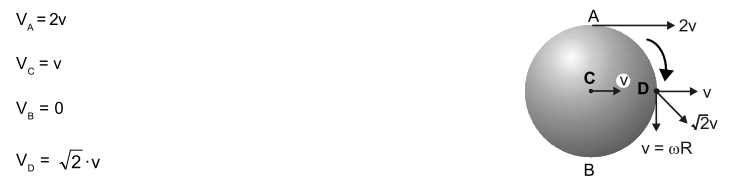ROLLING ON AINCLINED PLANE Let us consider the case of a rigid body of radius R and mass M rolling down without slipping on a smooth inclined plane having an angle of inclination $\theta$ as shown in fig. As the body rolls down(s) it suffer vertical descent (h) and therefore loses its potential energy. At the same time it acquires linear and angular speeds and hence, gains kinetic energy of translation and that of rotation. If there is no loss in total energy, then the loss of potential energy is equal to the gain in kinetic energy. $[\mathrm{A}] \quad$ With the help of law conservation of Mechenical Energy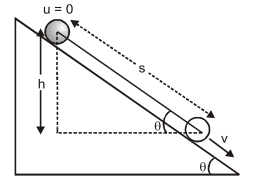$\mathrm{Mgh}=\frac{1}{2} \mathrm{Mv}^{2}+\frac{1}{2} \mathrm{I} \omega^{2} \quad\left(\mathrm{I}=\mathrm{Mk}^{2}, \quad \mathrm{v}=\omega \mathrm{R}\right)$ $\mathrm{Mgh}=\frac{1}{2} \mathrm{Mv}^{2}+\frac{1}{2} \mathrm{Mk}^{2}\left[\frac{\mathrm{v}^{2}}{\mathrm{R}^{2}}\right]$ $\mathrm{Mgh}=\frac{1}{2} \mathrm{Mv}^{2}\left[1+\frac{\mathrm{k}^{2}}{\mathrm{R}^{2}}\right]$ $v=\sqrt{\frac{2 g h}{1+\frac{k^{2}}{R^{2}}}}$   $[\mathrm{B}] \quad$ By Newton's equation of motion $\mathrm{v}^{2}=\mathrm{u}^{2}+2 \mathrm{as} \quad \mathrm{u}=0 \quad$ and $\quad \mathrm{a}=$ acceleration along inclined plane $\frac{2 \mathrm{gh}}{1+\frac{\mathrm{k}^{2}}{\mathrm{R}^{2}}}=2 \mathrm{as}$ $a=\frac{g \sin \theta}{1+\frac{k^{2}}{R^{2}}}$                                    $(\mathrm{h}=\mathrm{s} \sin \theta)$ $\mathbf{a}_{\text {rolling }}, \quad \mathbf{V}_{\text {rolling }}$ and $\mathbf{t}_{\text {rolling }}$ does not depends on the mass of Object,.   $[\mathrm{C}] \quad$ By Newton's $2^{\text {nd }} \mathrm{eq}^{\text {n }}$, of motion $\mathrm{s}=\mathrm{ut}+\frac{1}{2} \mathrm{at}^{2} \quad \mathrm{u}=0$ $\mathrm{t}=\sqrt{\frac{2 \mathrm{s}}{\mathrm{a}}}$ put the value of acceleration $t=\sqrt{\frac{2 s\left(1+\frac{k^{2}}{R^{2}}\right)}{g \sin \theta}}$\ For different shape of bodies if they roll down or inclined plane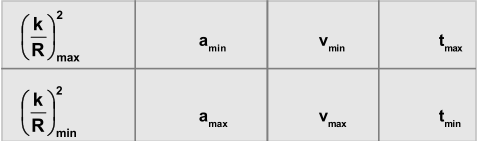Special Note: If body is sliding on inclined plane. Then \begin{aligned} \text { Then } & \frac{\mathrm{k}^{2}}{\mathrm{R}^{2}}=0 \\ & \mathrm{v}=\sqrt{2 \mathrm{gh}} \\ \mathrm{a} &=\mathrm{g} \sin \theta \\ \mathrm{t} &=\sqrt{\frac{2 \mathrm{s}}{\mathrm{g} \sin \theta}} \end{aligned}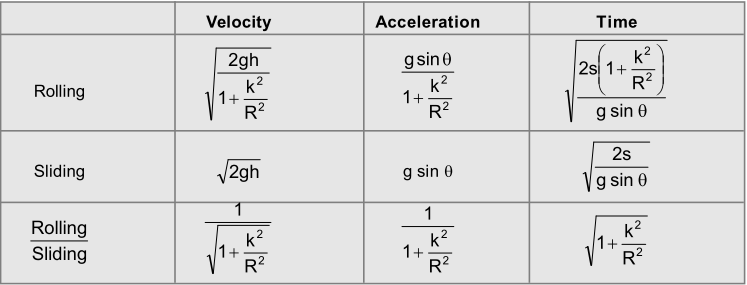The various forces acting on the rolling down body are: (i) The weight (Mg)ofthe booyin the vertically downward the body which action. (iii) Normal reaction(N)of the surface of the plane on the body which acts normal to the inclined plane. (iii) The force offiction ( $f$ ), which acts opposite to the direction of motion of the body. Mg $\sin \theta-f=M a$ Mg $\sin \theta-f=M\left[\frac{g \sin \theta}{1+k^{2} / R^{2}}\right]$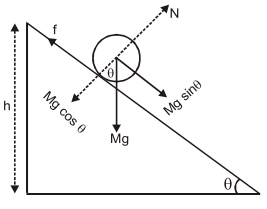$f=\operatorname{Mg} \sin \theta\left[1-\frac{1}{1+k^{2} / R^{2}}\right]$ $\mu[\mathrm{Mg} \cos \theta]=\mathrm{Mg} \sin \theta\left[\frac{\frac{\mathrm{k}^{2}}{\mathrm{R}^{2}}}{1+\frac{\mathrm{k}^{2}}{\mathrm{R}^{2}}}\right]$ $\mu=\frac{\tan \theta}{1+\frac{R^{2}}{k^{2}}}$                  [For disc and cylinder $\left.\frac{\mathrm{R}^{2}}{\mathrm{k}^{2}}=2\right]$         The condition for rolling motion of the cylinder along the inclined plane without slipping $\mu_{\mathrm{s}} \geq \frac{1}{3} \tan \theta$ Examples: Ex.1 When a sphere of moment of inertia 'I'rolls down an inclined plane then percentage of rotational kinetic energy to its total kinetic energy will be. ans. $\frac{E_{r}}{E_{T}} \times 100=\frac{\frac{1}{2} I \omega^{2}}{\frac{1}{2} m v^{2}+\frac{1}{2} I \omega^{2}} \times 100=\frac{\frac{2}{5} m r^{2} \times \frac{v^{2}}{r^{2}}}{m v^{2}\left(1+\frac{K^{2}}{r^{2}}\right)} \times 100=28 \%$ Ex. A solid sphere of mass $0.1 \mathrm{kg}$ and radius $20 \mathrm{cm} .$ rolls down an inclined $1.4 \mathrm{m}$. in length $$\left[\theta=\sin ^{-1}(1 / 10)\right] \text { starting from rest its final velocity will be. }$$ $v=\sqrt{\frac{2 g L \sin \theta}{1+\frac{K^{2}}{R^{2}}}}=\sqrt{\frac{2 \times 9.8 \times 1 \times 5 \times 1.4}{10 \times 7}}=1.4 \mathrm{m} / \mathrm{sec}$      $\sin \theta=\frac{1}{10} \quad \frac{\mathrm{K}^{2}}{\mathrm{r}^{2}}=\frac{2}{5}$     Introduction to Rotational Dynamics  Moment of Inertia Moment of Inertia: Perpendicular and Parallel axis theorem Radius of Gyration Law of Conservation of Angular Momentum Conservation of Angular Momentum Examples Kinetic Energy of a Rotating Body Work done in rotatory Motion Rotational Power Combine Translational and Rotational Motion Rolling without slipping Rolling on a plane surface Rolling on a Inclined Plane For Latest updates, Subscribe our Youtube Channel